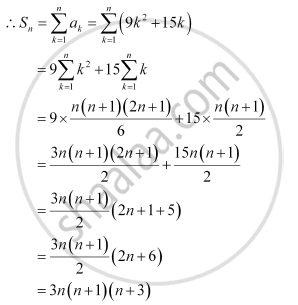Share

# Find the Sum to N Terms of the Series 3 × 8 + 6 × 11 + 9 × 14 +… - Mathematics

#### Question

Find the sum to n terms of the series 3 × 8 + 6 × 11 + 9 × 14 +…

#### Solution

The given series is 3 × 8 + 6 × 11 + 9 × 14 + …

a= (nth term of 3, 6, 9 …) × (nth term of 8, 11, 14, …)

= (3n) (3n + 5)

= 9n2 + 15nIs there an error in this question or solution?